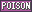# Types

Types refer to different elemental properties associated with both Pokémon and their moves. There are 18 total types of Pokémon: Normal, Fire, Water, Grass, Electric, Ice, Fighting, Poison, Ground, Flying, Psychic, Bug, Rock, Ghost, Dragon, Dark, Steel and Fairy. These 18 types apply to both Pokémon and their moves.

## Characteristics

Each type has its own strengths and weaknesses, most of which are logical setups. Fire-type Pokémon are weak to Water-type Pokémon, Ice-type Pokémon are weak to Fire-type Pokémon, Water-type Pokémon are weak to Electric-type Pokémon, etc. These matchups also apply to the types of moves as well. A Fire-type move such as Ember will be strong against a Grass-type Pokémon such as Bulbasaur.

There are also dual-type Pokémon, Pokémon that belong to two types. Most if not all dual-type Pokémon's types correspond with one another such as a Bug/Poison-type Pokémon or a Rock/Ground-type Pokémon. However, not all dual-type Pokémon's types relate to each other like this. Some Pokémon may be dual-type between two types that don't normally go together such as Water/Electric-types (such as Lanturn), Grass/Flying-types (such as Jumpluff) or Fighting/Psychic (such as Medicham). Pokémon themselves can have up to two types (making them dual-type Pokémon), but moves can only be one type.

## Chart

ATK
Type
DEF Type×1
×1
×1
×1
×1
×1
×1
×1
×1
×1
×1
×1
½
×0
×1
×1
½
×1×1
½
½
×2
×1
×2
×1
×1
×1
×1
×1
×2
½
×1
½
×1
×2
×1×1
×2
½
½
×1
×1
×1
×1
×2
×1
×1
×1
×2
×1
½
×1
×1
×1×1
½
×2
½
×1
×1
×1
½
×2
½
×1
½
×2
×1
½
×1
×1
×1×1
×1
×2
½
½
×1
×1
×1
×0
×2
×1
×1
×1
×1
½
×1
×1
×1×1
½
½
×2
×1
½
×1
×1
×2
×2
×1
×1
×1
×1
×2
×1
½
×1×2
×1
×1
×1
×1
×1
×1
½
×1
½
½
½
×2
×0
×1
×2
×2
×1×1
×1
×1
×2
×1
×1
×1
½
½
×1
×1
×1
½
½
×1
×1
×0
×1×1
×2
×1
×1
×2
×1
×1
×2
×1
×0
×1
×1
×2
×1
×1
×1
×2
×1×1
×1
×1
×2
½
×1
×2
×1
×1
×1
×1
×2
½
×1
×1
×1
½
×1×1
×1
×1
×1
×1
×1
×2
×2
×1
×1
½
×1
×1
×1
×1
×0
½
×1×1
½
×1
×2
×1
×1
½
½
×1
½
×2
×1
×1
½
×1
×2
½
×1×1
×2
×1
×1
×1
×2
½
×1
½
×2
×1
×2
×1
×1
×1
×1
½
×1×0
×1
×1
×1
×1
×1
×1
×1
×1
×1
×2
×1
×1
×2
×1
½
½
×1×1
×1
×1
×1
×1
×1
×1
×1
×1
×1
×1
×1
×1
×1
×2
×1
½
×1×1
×1
×1
×1
×1
×1
½
×1
×1
×1
×2
×1
×1
×2
×1
½
½
×1×1
½
½
×1
½
×2
×1
×1
×1
×1
×1
×1
×2
×1
×1
×1
½
×1×1
×1
×1
×1
×1
×1
×1
×1
×1
×1
×1
×1
×1
×1
×2
×1
×1
×1
×2
Super effective
×1
Normal
½
Resistant
×0
No damage